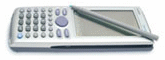2 replies to this topic

### #1hasan_hzl

hasan_hzl

Newbie

•• Members
•• 2 posts

Posted 07 June 2009 - 06:05 PM

Hi,

I have been using my Classpad 300PLUS for maybe 2 years. Although I many times used solve option of it, last day it did not work. I wrote the following into classpad:

solve(sin(x)=1,x)

But it gave me a solution like

{x=360constn(1)+90}

But i don't want a solution like this. I want x=90 when it is in degree format. Also my calculator gives same kind of different results for equations including sin, cos, tan...

### #2Lovecasio

Lovecasio

Casio Freak

•• Members
•• 242 posts
• Location:Hochiminh city Vietnam
• Interests:Organic chemistry.<br />Pharmacy

• Calculators:
fx 570 MS, Casio AFX 2.0+, ClassPad 300

Posted 08 June 2009 - 01:06 PM

It is completely right solution. constn(1) means any integer number. As we know the solution for sin(x)=1 is x=pi/2 + 2kpi. k here equal constn(1).
If you want to have only one specific solution, try solve(sin(x)=1,x)|0<x<180
Good luck. and welcome to the forum### #3hasan_hzl

hasan_hzl

Newbie

•• Members
•• 2 posts

Posted 10 June 2009 - 05:27 AM

It is completely right solution. constn(1) means any integer number. As we know the solution for sin(x)=1 is x=pi/2 + 2kpi. k here equal constn(1).
If you want to have only one specific solution, try solve(sin(x)=1,x)|0<x<180
Good luck. and welcome to the forumThis is correct I agree. But it also gives solution of the following equation for M in terms of tan(M^2). It has to iterate and give a solution for M. A number:) Not a olution like M = tan(M^2 bla bla...) When I write solve(equation,M,0) it gives correct one, i mean two M values. In the past it was not like this.#### 0 user(s) are reading this topic

0 members, 0 guests, 0 anonymous users